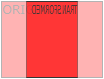THE WORLD'S LARGEST WEB DEVELOPER SITE

CSS 3D Transforms

CSS 3D Transforms

CSS also supports 3D transformations.

Mouse over the elements below to see the difference between a 2D and a 3D transformation:

2D rotate
3D rotate

In this chapter you will learn about the following CSS property:

• transform

Browser Support

The numbers in the table specify the first browser version that fully supports the property.

Property
transform 36.0
10.0
16.0
9.0
23.0

Browser Specific Prefixes

Some older browsers need specific prefixes (-webkit-) to understand the 3D transform properties:

Example

#myDiv {
-webkit-transform: rotateY(130deg); /* Safari prior 9.0 */
transform: rotateY(130deg); /* Standard syntax */
}
Try it Yourself »

CSS 3D Transforms Methods

With the CSS transform property you can use the following 3D transformation methods:

• rotateX()
• rotateY()
• rotateZ()

The rotateX() MethodThe rotateX() method rotates an element around its X-axis at a given degree:

Example

#myDiv {
transform: rotateX(150deg);
}
Try it Yourself »

The rotateY() MethodThe rotateY() method rotates an element around its Y-axis at a given degree:

Example

#myDiv {
transform: rotateY(130deg);
}
Try it Yourself »

The rotateZ() Method

The rotateZ() method rotates an element around its Z-axis at a given degree:

Example

#myDiv {
transform: rotateZ(90deg);
}
Try it Yourself »

CSS Transform Properties

The following table lists all the 3D transform properties:

Property Description
transform Applies a 2D or 3D transformation to an element
transform-origin Allows you to change the position on transformed elements
transform-style Specifies how nested elements are rendered in 3D space
perspective Specifies the perspective on how 3D elements are viewed
perspective-origin Specifies the bottom position of 3D elements
backface-visibility Defines whether or not an element should be visible when not facing the screen

CSS 3D Transform Methods

Function Description
matrix3d
(n,n,n,n,n,n,n,n,n,n,n,n,n,n,n,n)
Defines a 3D transformation, using a 4x4 matrix of 16 values
translate3d(x,y,z) Defines a 3D translation
translateX(x) Defines a 3D translation, using only the value for the X-axis
translateY(y) Defines a 3D translation, using only the value for the Y-axis
translateZ(z) Defines a 3D translation, using only the value for the Z-axis
scale3d(x,y,z) Defines a 3D scale transformation
scaleX(x) Defines a 3D scale transformation by giving a value for the X-axis
scaleY(y) Defines a 3D scale transformation by giving a value for the Y-axis
scaleZ(z) Defines a 3D scale transformation by giving a value for the Z-axis
rotate3d(x,y,z,angle) Defines a 3D rotation
rotateX(angle) Defines a 3D rotation along the X-axis
rotateY(angle) Defines a 3D rotation along the Y-axis
rotateZ(angle) Defines a 3D rotation along the Z-axis
perspective(n) Defines a perspective view for a 3D transformed element